# MITx: 15.071x The Analytics Edge

### Predicting loan repayment and Risk

In the lending industry, investors provide loans to borrowers in exchange for the promise of repayment with interest. If the borrower repays the loan, then the lender profits from the interest. However, if the borrower is unable to repay the loan, then the lender loses money. Therefore, lenders face the problem of predicting the risk of a borrower being unable to repay a loan.

### 1. Preparing the Dataset

Load the dataset loans.csv into a data frame called loans, and explore it using the str() and summary() functions. What proportion of the loans in the dataset were not paid in full? Please input a number between 0 and 1.

• ANS: 0.1600543

Explanation
From table(loans$not.fully.paid), we see that 1533 loans were not paid, and 8045 were fully paid. Therefore, the proportion of loans not paid is 1533/(1533+8045)=0.1601. Using a revised version of the dataset that has the missing values filled in with multiple imputation. ### 2. Prediction Models 1. Now that we have prepared the dataset, we need to split it into a training and testing set. To ensure everybody obtains the same split, set the random seed to 144 (even though you already did so earlier in the problem) and use the sample.split function to select the 70% of observations for the training set (the dependent variable for sample.split is not.fully.paid). Name the data frames train and test. Now, use logistic regression trained on the training set to predict the dependent variable not.fully.paid using all the independent variables. • Which independent variables are significant in our model? (Significant variables have at least one star, or a Pr(>|z|) value less than 0.05.) Select all that apply. Variables that are significant have at least one star in the coefficients table of the summary(model0) output. Note that some have a positive coefficient (meaning that higher values of the variable lead to an increased risk of defaulting) and some have a negative coefficient (meaning that higher values of the variable lead to a decreased risk of defaulting). 2. Consider two loan applications, which are identical other than the fact that the borrower in Application A has FICO credit score 700 while the borrower in Application B has FICO credit score 710. Let Logit(A) be the log odds of loan A not being paid back in full, according to our logistic regression model, and define Logit(B) similarly for loan B. • Logit = belta'*x • Logit(A) - Logit(B) = belta'*(x(A)-x(B)) • What is the value of Logit(A) - Logit(B)? • ANS: 0.09406 Now, let O(A) be the odds of loan A not being paid back in full, according to our logistic regression model, and define O(B) similarly for loan B. What is the value of O(A)/O(B)? (HINT: Use the mathematical rule that exp(A + B + C) = exp(A)exp(B)exp(C). Also, remember that exp() is the exponential function in R.) • What is the value of O(A)/O(B)? • ANS: 1.0986257 ### 3. Prediction Models 1. Predict the probability of the test set loans not being paid back in full (remember type="response" for the predict function). Store these predicted probabilities in a variable named predicted.risk and add it to your test set (we will use this variable in later parts of the problem). Compute the confusion matrix using a threshold of 0.5. What is the accuracy of the logistic regression model? Input the accuracy as a number between 0 and 1. • What is the accuracy of the logistic regression model? • ANS: 0.8364079 • What is the accuracy of the baseline model? • ANS: 0.8398886 Explanation The confusion matrix can be computed with the following commands: test$predicted.risk = predict(mod, newdata=test, type="response") table(test$not.fully.paid, test$predicted.risk > 0.5)
2403 predictions are correct (accuracy 2403/2873=0.8364), while 2413 predictions would be correct in the baseline model of guessing every loan would be paid back in full (accuracy 2413/2873=0.8399).

2. Use the ROCR package to compute the test set AUC.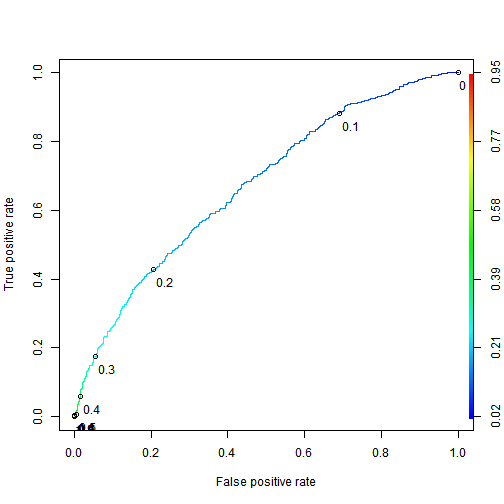• ANS: 0.6720995

Explanation
The test set AUC can be computed with the following commands: library(ROCR) pred = prediction(test$predicted.risk, test$not.fully.paid) as.numeric(performance(pred, "auc")@y.values)

The model has poor accuracy at the threshold 0.5. But despite the poor accuracy, we will see later how an investor can still leverage this logistic regression model to make profitable investments.

### 4. A "Smart Baseline"

1. In the previous problem, we built a logistic regression model that has an AUC significantly higher than the AUC of 0.5 that would be obtained by randomly ordering observations. However, LendingClub.com assigns the interest rate to a loan based on their estimate of that loan's risk. This variable, int.rate, is an independent variable in our dataset. In this part, we will investigate using the loan's interest rate as a "smart baseline" to order the loans according to risk. Using the training set, build a bivariate logistic regression model (aka a logistic regression model with a single independent variable) that predicts the dependent variable not.fully.paid using only the variable int.rate. The variable int.rate is highly significant in the bivariate model, but it is not significant at the 0.05 level in the model trained with all the independent variables. What is the most likely explanation for this difference?

• ANS: int.rate is correlated with other risk-related variables, and therefore does not incrementally improve the model when those other variables are included.

Explanation
To train the bivariate model, run the following command: bivariate = glm(not.fully.paid~int.rate, data=train, family="binomial") summary(bivariate)

Decreased significance between a bivariate and multivariate model is typically due to correlation. From cor(train$int.rate, train$fico), we can see that the interest rate is moderately well correlated with a borrower's credit score.

Training/testing set split rarely has a large effect on the significance of variables (this can be verified in this case by trying out a few other training/testing splits), and the models were trained on the same observations.

2. Make test set predictions for the bivariate model. What is the highest predicted probability of a loan not being paid in full on the testing set?

• ANS: 0.4266
• With a logistic regression cutoff of 0.5, how many loans would be predicted as not being paid in full on the testing set?
• ANS: 0

Explanation
Make and summarize the test set predictions with the following code:

pred.bivariate = predict(bivariate, newdata=test, type="response")

summary(pred.bivariate)

According to the summary function, the maximum predicted probability of the loan not being paid back is 0.4266, which means no loans would be flagged at a logistic regression cutoff of 0.5.

3. What is the test set AUC of the bivariate model?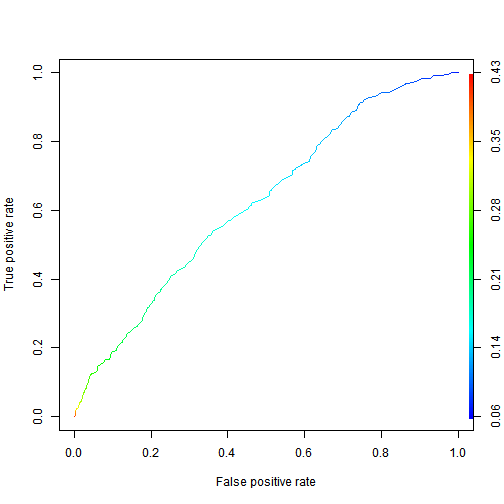• ANS: 0.6239081

Explanation
The AUC can be computed with: prediction.bivariate = prediction(pred.bivariate, test$not.fully.paid) as.numeric(performance(prediction.bivariate, "auc")@y.values) ### 5. Computing the Profitability of an Investment While thus far we have predicted if a loan will be paid back or not, an investor needs to identify loans that are expected to be profitable. If the loan is paid back in full, then the investor makes interest on the loan. However, if the loan is not paid back, the investor loses the money invested. Therefore, the investor should seek loans that best balance this risk and reward. To compute interest revenue, consider a$c investment in a loan that has an annual interest rate r over a period of t years. Using continuous compounding of interest, this investment pays back c * exp(rt) dollars by the end of the t years, where exp(rt) is e raised to the r*t power.
How much does a $10 investment with an annual interest rate of 6% pay back after 3 years, using continuous compounding of interest? Hint: remember to convert the percentage to a proportion before doing the math. Enter the number of dollars, without the$ sign.

$$c^(r*t)$$

Explanation
In this problem, we have c=10, r=0.06, and t=3. Using the formula above, the final value is 10exp(0.063) = 11.97.

In the previous subproblem, we concluded that an investor who invested c dollars in a loan with interest rate r for t years makes c * (exp(rt) - 1) dollars of profit if the loan is paid back in full and -c dollars of profit if the loan is not paid back in full (pessimistically). In order to evaluate the quality of an investment strategy, we need to compute this profit for each loan in the test set. For this variable, we will assume a $1 investment (aka c=1). To create the variable, we first assign to the profit for a fully paid loan, exp(rt)-1, to every observation, and we then replace this value with -1 in the cases where the loan was not paid in full. All the loans in our dataset are 3-year loans, meaning t=3 in our calculations. Enter the following commands in your R console to create this new variable: • What is the maximum profit of a$10 investment in any loan in the testing set (do not include the $sign in your answer)? • ANS 8.8947687 Explanation From summary(test$profit), we see the maximum profit for a $1 investment in any loan is$0.8895. Therefore, the maximum profit of a $10 investment is 10 times as large, or$8.895. summary(test$profit) ### 6. An Investment Strategy Based on Risk 1. A simple investment strategy of equally investing in all the loans would yield profit$20.94 for a $100 investment. But this simple investment strategy does not leverage the prediction model we built earlier in this problem. As stated earlier, investors seek loans that balance reward with risk, in that they simultaneously have high interest rates and a low risk of not being paid back. To meet this objective, we will analyze an investment strategy in which the investor only purchases loans with a high interest rate (a rate of at least 15%), but amongst these loans selects the ones with the lowest predicted risk of not being paid back in full. We will model an investor who invests$1 in each of the most promising 100 loans. First, use the subset() function to build a data frame called highInterest consisting of the test set loans with an interest rate of at least 15%.
What is the average profit of a $1 investment in one of these high-interest loans (do not include the$ sign in your answer)?

• ANS: 0.2251015

What proportion of the high-interest loans were not paid back in full?

• ANS: 0.2517162

Explanation
The following two commands build the data frame highInterest and summarize the profit variable. highInterest = subset(test, int.rate >= 0.15) summary(highInterest$profit) We read that the mean profit is$0.2251. To obtain the breakdown of whether the loans were paid back in full, we can use table(highInterest$not.fully.paid) 110 of the 437 loans were not paid back in full, for a proportion of 0.2517. 2. Next, we will determine the 100th smallest predicted probability of not paying in full by sorting the predicted risks in increasing order and selecting the 100th element of this sorted list. Find the highest predicted risk that we will include by typing the following command into your R console: Use the subset() function to build a data frame called selectedLoans consisting of the high-interest loans with predicted risk not exceeding the cutoff we just computed. Check to make sure you have selected 100 loans for investment. What is the profit of the investor, who invested$1 in each of these 100 loans (do not include the \$ sign in your answer)?

• ANS: 31.2782493

How many of 100 selected loans were not paid back in full?

• ANS: 19

# R Test

### R Markdown...

This is an R Markdown document. Markdown is a simple formatting syntax for authoring HTML, PDF, and MS Word documents. For more details on using R Markdown see http://rmarkdown.rstudio.com.

When you click the Knit button a document will be generated that includes both content as well as the output of any embedded R code chunks within the document. You can embed an R code chunk like this:

summary(cars)

##      speed           dist
##  Min.   : 4.0   Min.   :  2.00
##  1st Qu.:12.0   1st Qu.: 26.00
##  Median :15.0   Median : 36.00
##  Mean   :15.4   Mean   : 42.98
##  3rd Qu.:19.0   3rd Qu.: 56.00
##  Max.   :25.0   Max.   :120.00


You can also embed plots, for example: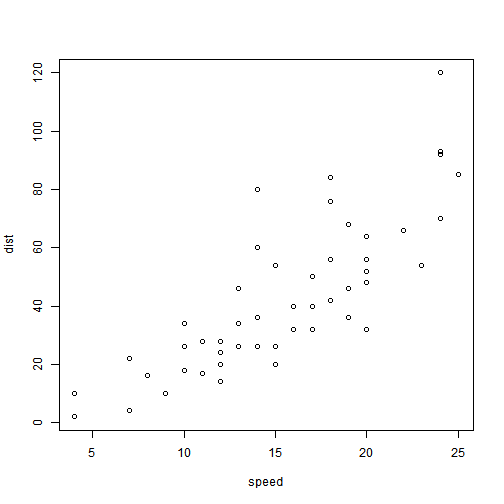Note that the echo = FALSE parameter was added to the code chunk to prevent printing of the R code that generated the plot.

# What's Jekyll?

Jekyll is a static site generator, an open-source tool for creating simple yet powerful websites of all shapes and sizes. From the project's readme:

Jekyll is a simple, blog aware, static site generator. It takes a template directory [...] and spits out a complete, static website suitable for serving with Apache or your favorite web server. This is also the engine behind GitHub Pages, which you can use to host your project’s page or blog right here from GitHub.

It's an immensely useful tool and one we encourage you to use here with Hyde.

Find out more by visiting the project on GitHub.

# Analysis on U.S. NOAA storm database

### Synopsis

Storms and other severe weather events can cause both public health and economic problems for communities and municipalities. Many severe events can result in fatalities, injuries, and property damage, and preventing such outcomes to the extent possible is a key concern.

The basic goal of this assignment is to explore the NOAA Storm Database and answer some basic questions about severe weather events. The events in the database start in the year 1950 and end in November 2011.

1. Across the United States, which types of events (as indicated in the EVTYPE variable) are most harmful with respect to population health?

2. Across the United States, which types of events have the greatest economic consequences?

## Results

• In this study, it's assumed that harmful events with respect to population health comes from variables FATALITIES and INJURIES.

• Select useful data

• Create new variables: TOTALPROPDMG, TOTALCROPDMG and TOTALDMG with: TOTALDMG = (TOTALPROPDMG + TOTALCROPDMG)

### Population health impact

1. Across the United States, which types of events (as indicated in the EVTYPE variable) are most harmful with respect to population health?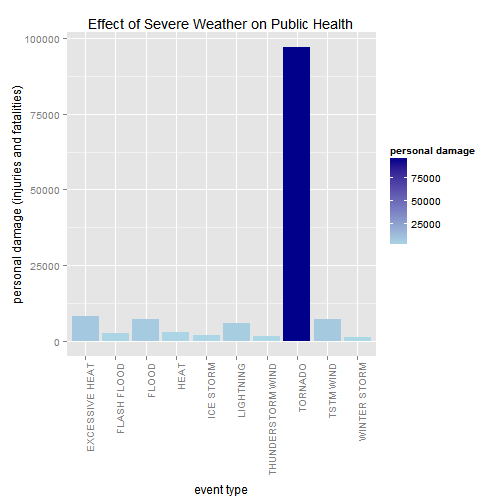• From the above figure we can see that TORNADOES have the most significant impact on public health.

2. Across the United States, which types of events have the greatest economic consequences?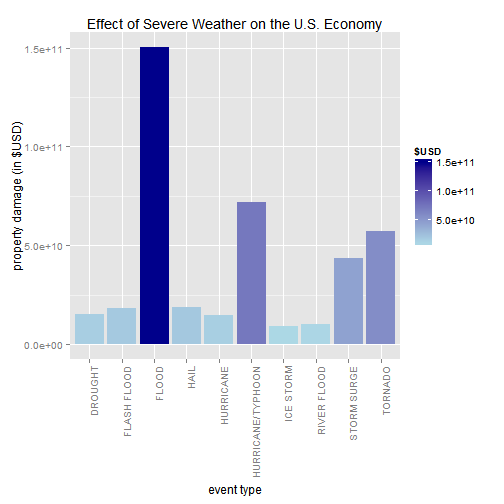• The FLOODS, HURRICANES/TYPHOONES and TORNADOES are the events with the greatest economic consequences.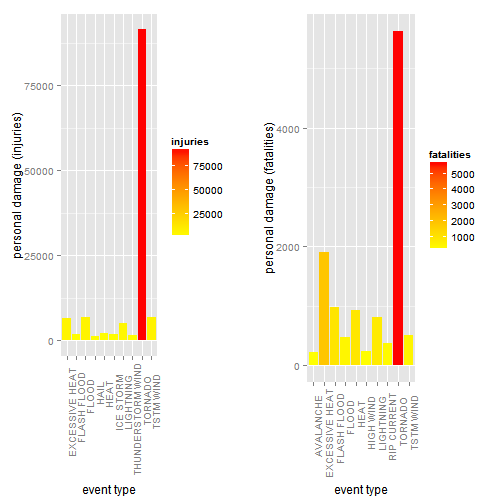• TORNADO is the harmful event with respect to population health, and
• FLOOD is the event which have the greatest economic consequences.

# Statistical Inference

## Introduction

This is the project for the statistical inference class. In it, you will use simulation to explore inference and do some simple inferential data analysis. The project consists of two parts:

1. A simulation exercise.
2. Basic inferential data analysis.

## Simulation exercises

The exponential distribution can be simulated in R with rexp(n, lambda) where lambda is the rate parameter. The mean of exponential distribution is 1/lambda and the standard deviation is also 1/lambda. Set lambda = 0.2 for all of the simulations. You will investigate the distribution of averages of 40 exponentials. Note that you will need to do a thousand simulations.

## Results

### 1. Show the sample mean and compare it to the theoretical mean of the distribution.

The theoretical mean and sample mean for the exponential distribution.

Theoretical mean: 5

Sampling mean: 4.9644306

The sample mean is very close to the theoretical mean at 5.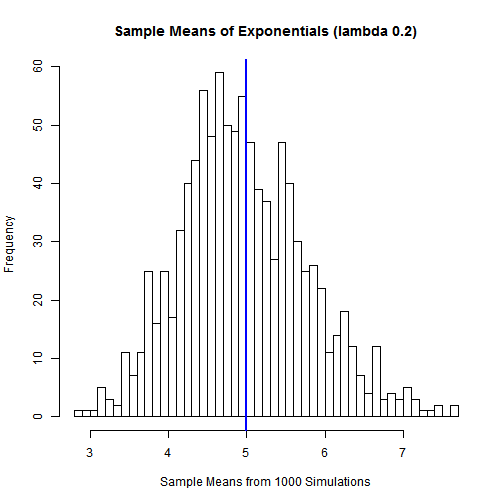### 2. Show how variable the sample is (via variance) and compare it to the theoretical variance of the distribution.

The variance for the sample data is : 0.6456619 and the theoretical variance is : 0.625 Both these values are quite close to each other.

### 3. Show that the distribution is approximately normal.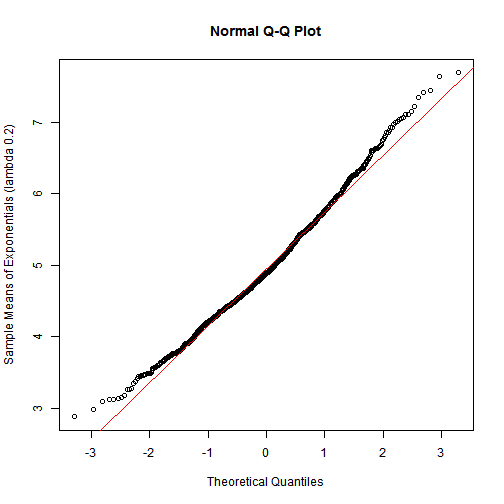we see that the distribution is approximately normal as the straight line is closer to the points.

# You're up and running!

Next you can update your site name, avatar and other options using the _config.yml file in the root of your repository (shown below).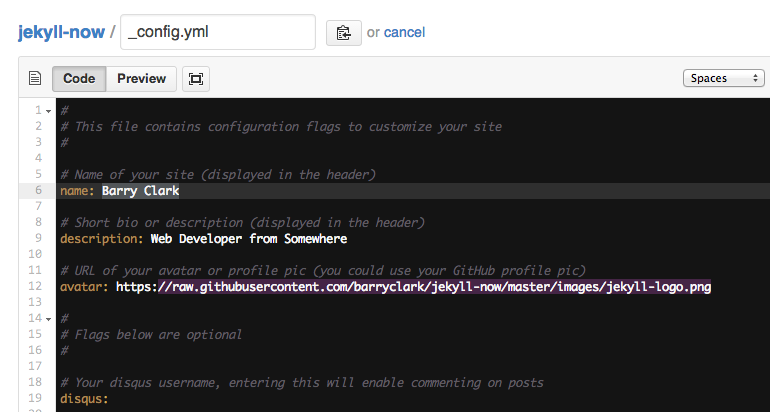The easiest way to make your first post is to edit this one. Go into /_posts/ and update the Hello World markdown file. For more instructions head over to the Jekyll Now repository on GitHub.